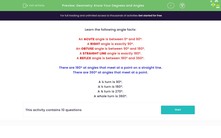# Understand Simple Angle Rules in GeometryKey stage:  KS 2

Curriculum topic:   Geometry: Properties of Shapes

Curriculum subtopic:   Know Angles and Degrees

Popular topics:   Geometry worksheets

Difficulty level:#### Worksheet Overview

Learn the following angle facts:

An ACUTE angle is between 0º and 90º.

A RIGHT angle is exactly 90º.

An OBTUSE angle is between 90º and 180º.

A STRAIGHT LINE angle is exactly 180º.

A REFLEX angle is between 180º and 360º.

There are 180º at angles that meet at a point on a straight line.

There are 360º at angles that meet at a point.

A ¼ turn is 90º.

A ½ turn is 180º.

A ¾ turn is 270º.

A whole turn is 360º.

### What is EdPlace?

We're your National Curriculum aligned online education content provider helping each child succeed in English, maths and science from year 1 to GCSE. With an EdPlace account you’ll be able to track and measure progress, helping each child achieve their best. We build confidence and attainment by personalising each child’s learning at a level that suits them.

Get started